# Electronics and Communication Engineering - Automatic Control Systems

### Exercise :: Automatic Control Systems - Section 2

41.

Assertion (A): Positive feedback is not used in any circuit.

Reason (R): Positive feedback leads to instability.

 A. Both A and R are correct and R is correct explanation of A B. Both A and R are correct but R is not correct explanation of A C. A is correct but R is wrong D. R is correct but A is wrong

Explanation:

Positive feedback is used in oscillator circuits.

42.

For the equation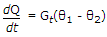where Q is heat energy Gt is thermal conductance and θ is temperature, analogous electrical equation is

 A. i = G(v1 - v2) B.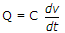C.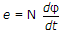D.Explanation:

Temperatures θ1 and θ2 are analogous to voltage v1 and v2 .

43.

If the Nyquist plot does not encircle the (-1 + j0) point the system is always stable.

 A. True B. False

Explanation:

It is not necessary.

44.

In op-amp circuit of the given figure, Eo(s)/Ei(s) =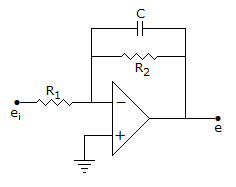A.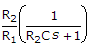B.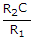C.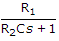D.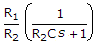Explanation: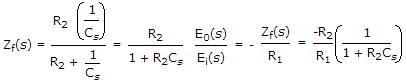45.

A system has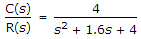For unit step input the settling time for 2% tolerance band is

 A. 1.6 B. 2.5 C. 4 D. 5

ξωn - 0.8 and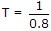= 1.25 s. For 2% criterion, settling time = 4T = 5 seconds.